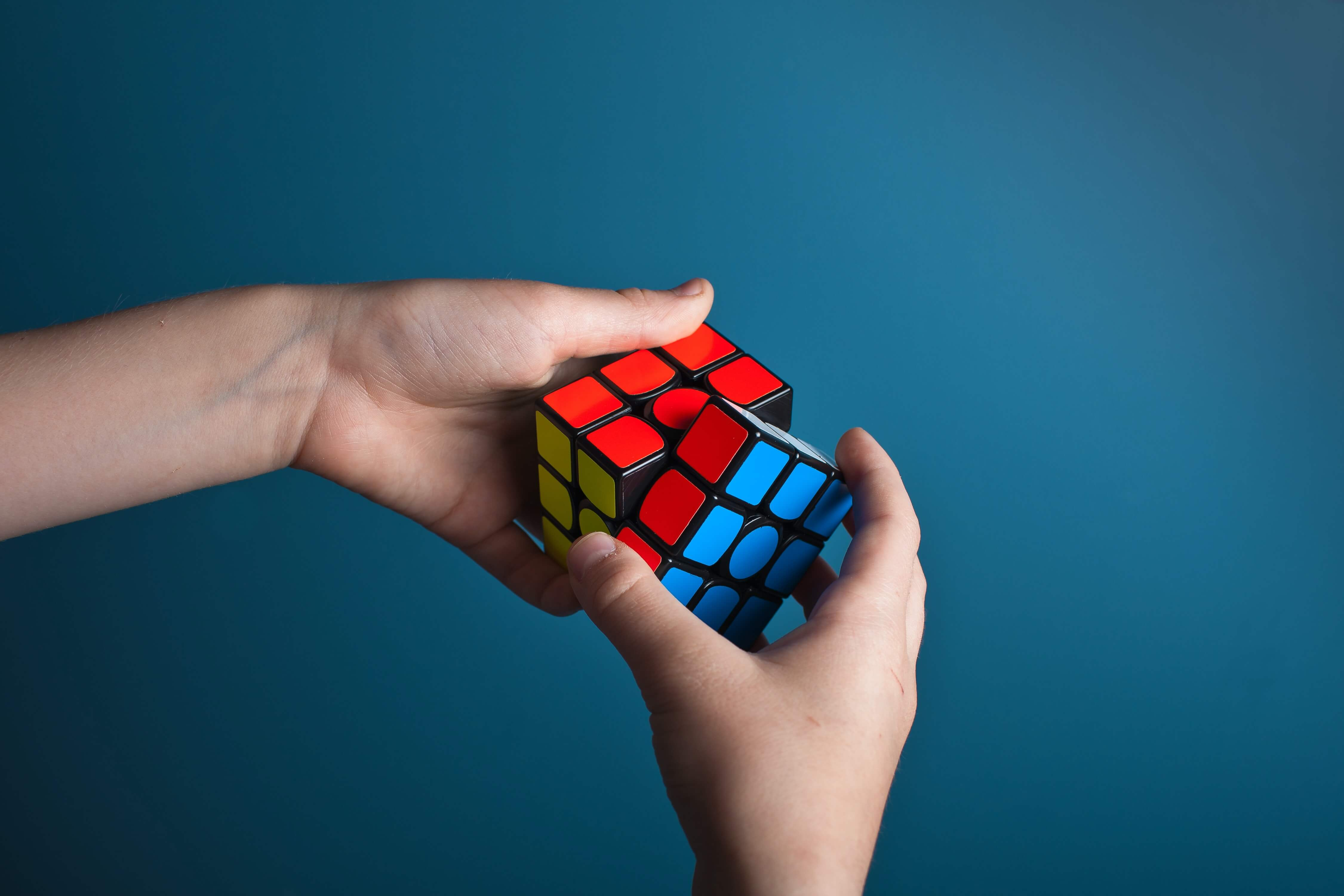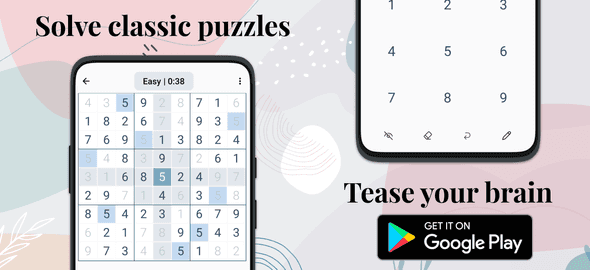# How many sudoku combinations exist?

There are a vast number of possible Sudoku puzzles that can be created, as there are many different ways to fill in the grid with the given constraints.

To determine the number of possible combinations, we need to consider the number of possible ways to fill in each cell of the grid. In a standard Sudoku puzzle, there are 9 rows, 9 columns, and 9 squares, and each cell must be filled with a number from 1 to 9. This means that there are 9 possible values that can be placed in each cell, and since there are 81 cells in the grid, there are a total of 9^81 possible combinations of values that can be placed in the grid.

This is a very large number, much too large to calculate or even express in standard notation. To give you an idea of just how large it is, if you were to try to write out all of the possible combinations, it would take more than 100 billion pages of text, assuming that each combination can be written on a single line.

To give you an idea of the size of this number, it is much larger than the number of atoms in the observable universe, which is estimated to be around 10^80. It is also much larger than the number of possible chess positions, which is estimated to be around 10^40.

To put it simply, the number of possible Sudoku combinations is extremely large and cannot be accurately expressed using standard notation. It is a number that is beyond our ability to fully comprehend or even express in a meaningful way

# Can there be multiple solutions to one sudoku puzzle?

Yes, it is possible for a Sudoku puzzle to have multiple solutions. In fact, most Sudoku puzzles are designed to have a unique solution, but it is possible to create puzzles that have multiple solutions.

A Sudoku puzzle is considered to have a unique solution if there is only one possible way to fill in the grid that satisfies the constraints of the puzzle. These constraints include the requirement that each row, column, and square must contain the numbers 1 through 9, and that no two cells in the same row, column, or square can contain the same number.

However, it is possible to create a Sudoku puzzle that has multiple solutions by leaving out certain cells or by including extra constraints that allow for more than one valid solution. These types of puzzles are known as "multi-solution" or "overdetermined" Sudoku puzzles.

In general, most Sudoku puzzles are designed to have a unique solution, but it is possible to create puzzles that have multiple solutions.